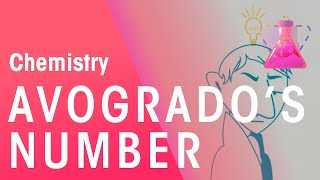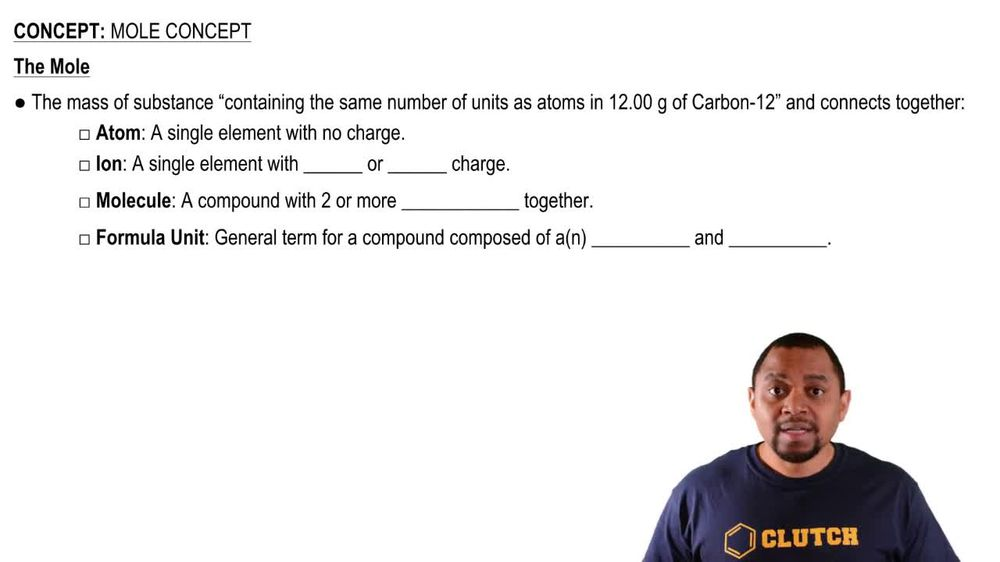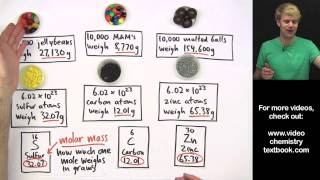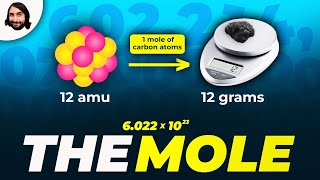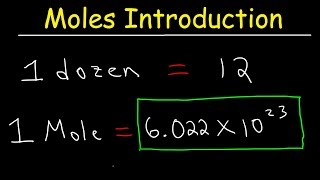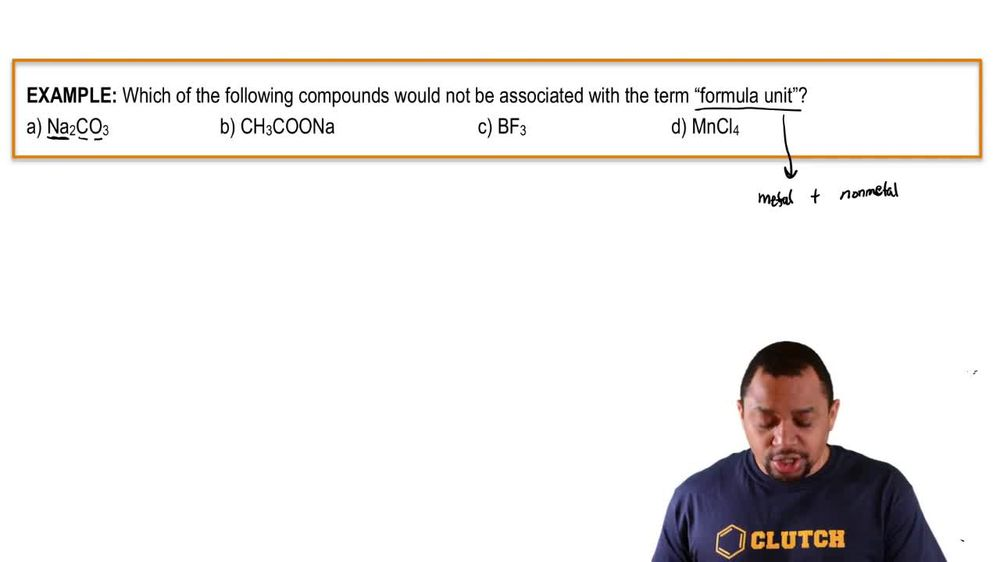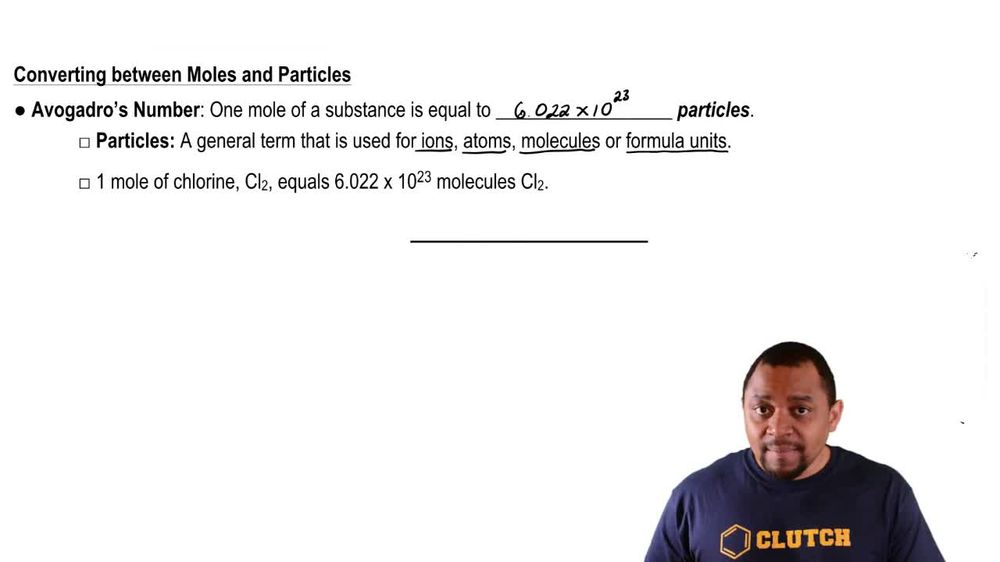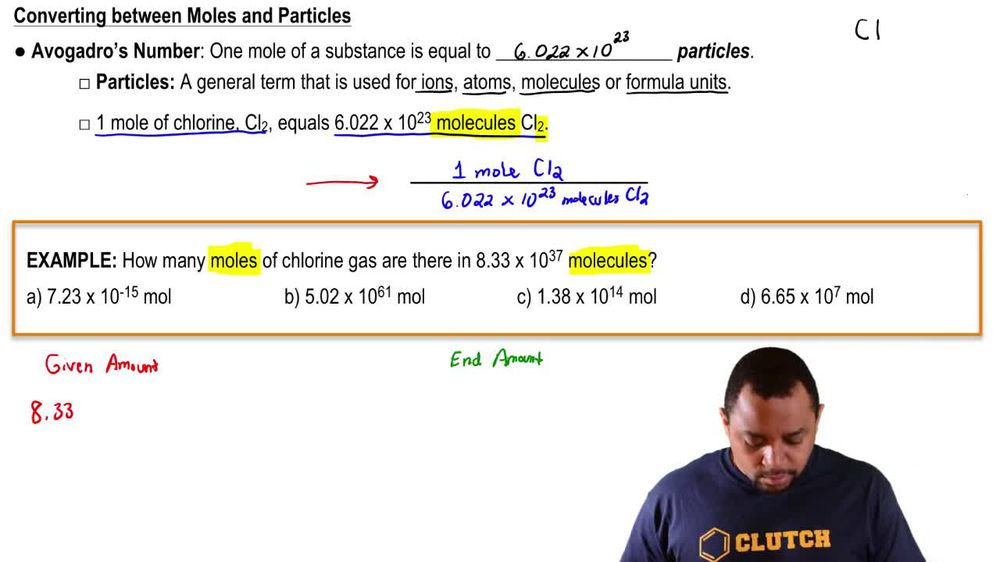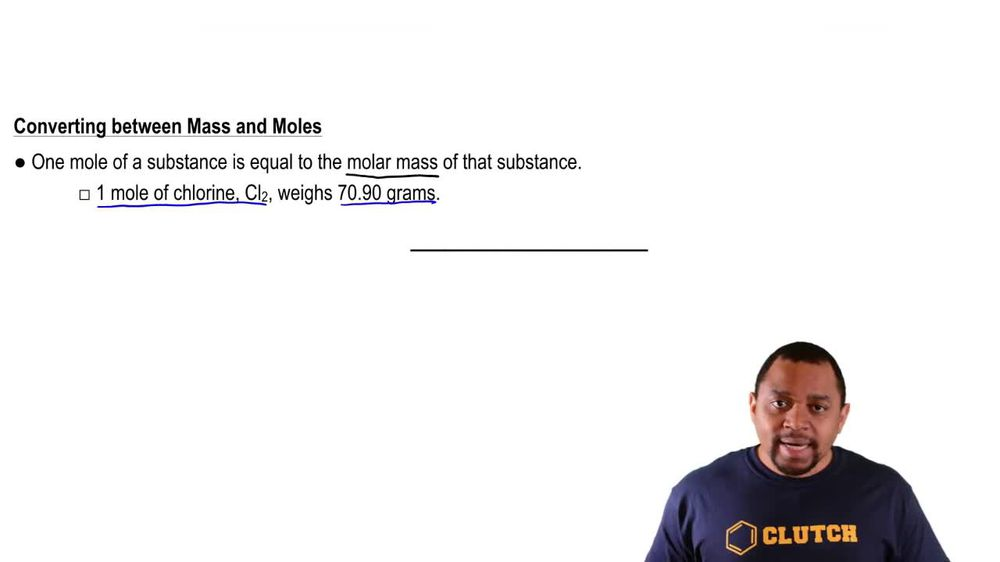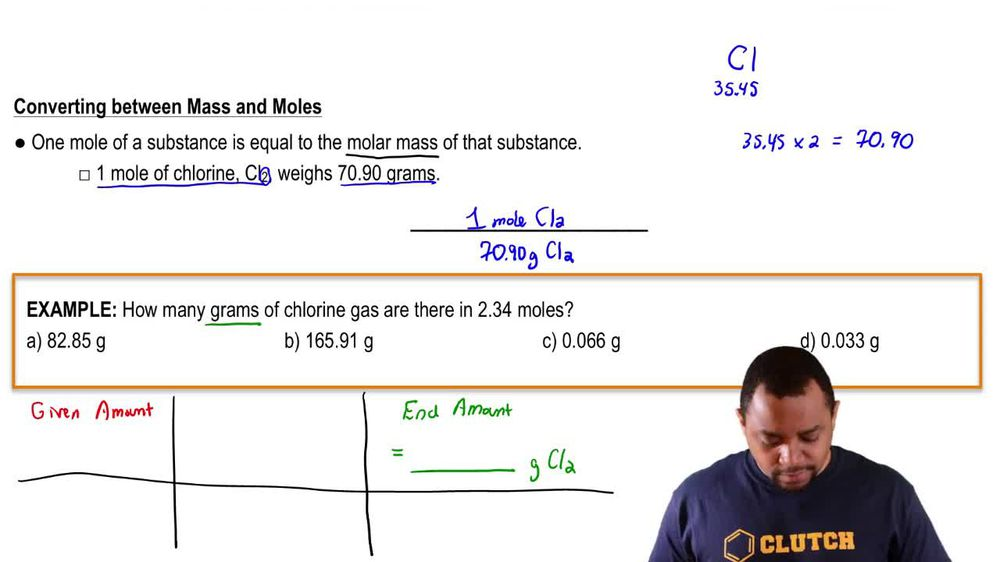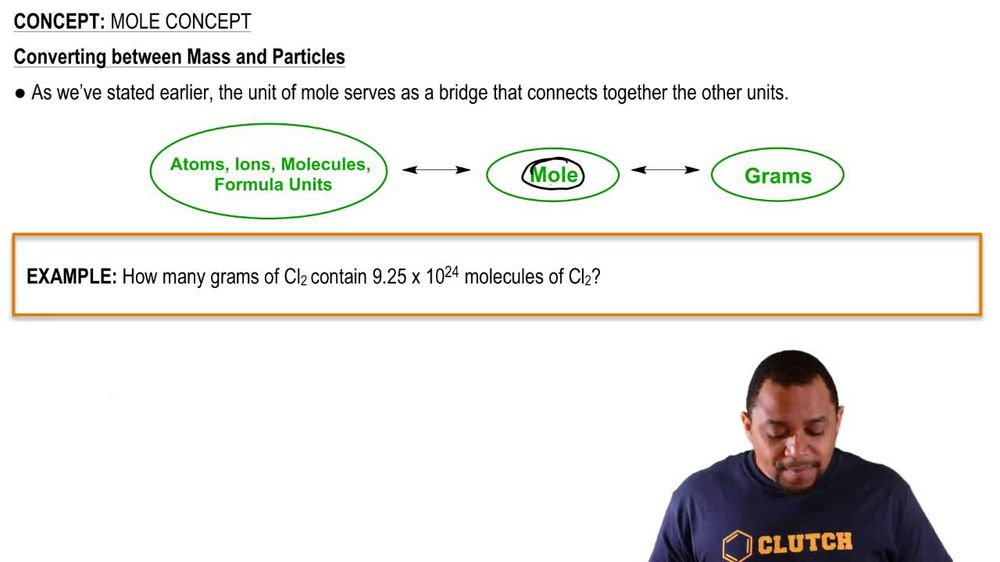Start typing, then use the up and down arrows to select an option from the list.
1. 2. Atoms & Elements2. Mole Concept
Problem

# Calculate the number of atoms in each sample. a. 5.18 g P

Relevant Solution2m
Play a video:
Hey everyone welcome back. So let's get started with this video. So here they want us to calculate the number of silver atoms and 4.62 g of silver. Okay, so here we're going to start with the given which is 4.62 g. So we're starting with g and we're going to go to malls. And how are we going to go to most? We're going to use the atomic mass of silver, which you can find on the periodic table and it's 107 0.87 grams per mole. Okay, But we want silver atoms. So for moles, we're going to go to atoms And how do we go for most to atoms where we're going to use avocados number, which is 6.02, 2 times 10 to the 23. Okay, so with this being said, Let's go ahead and start start our stock geometry. So let's start with the given which we said was Graham. So 4.62 grams of silver, which is a g. Okay, and then we said from grass grams to go to moles, we're going to use the atomic mass, which is 107 .87 g of silver and one mole of silver. Okay, so then we did this part. So not to go to most atoms. We're going to use avocados number. So then one mole of silver Is equal to 6.02, 2 times 10 to the 23 silver atoms, which is what we're looking for. So then we cancel out grams of silver molds of silver and we get Adams of silver, which is what we're looking for. We do the math and we get two points 58 Times 10 to the 22 silver atoms. Okay, Thank you for watching. I hope this helped, and I'll see you in the next video.